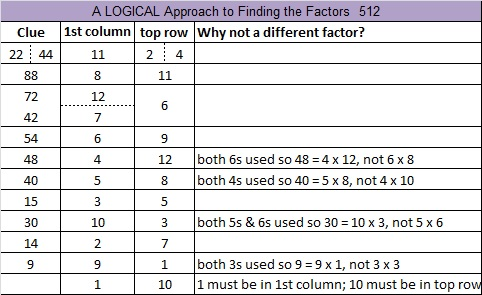# 512 Ants and Level 4The 512 Ants on Sullivan Street is an entertaining book for children that also includes learning activities by Marilyn Burns. The book teaches the important concept of doubling in a very natural way, but it can teach even more than that.  Here is a place value lesson for third grade students that uses the same book. There are relationships between adding, subtracting, doubling, multiplying, dividing, powers, and place value. The book and the place value lesson will make some of those relationships clear.

512 is a perfect cube because 512 = 8^3. It is also a cube of a cube because 8 = 2^3. Thus 512 = (2^3)^3 = 2^9. All of the factors of 512 are powers of 2.Print the puzzles or type the solution on this excel file: 12 Factors 2015-06-01

—————————————————————————————————

• 512 is a composite number.
• Prime factorization: 512 = 2 x 2 x 2 x 2 x 2 x 2 x 2 x 2 x 2, which can be written 512 = (2^9)
• The exponent in the prime factorization is 9. Adding one we get (9 + 1) = 10. Therefore 512 has exactly 10 factors.
• Factors of 512: 1, 2, 4, 8, 16, 32, 64, 128, 256, 512
• Factor pairs: 512 = 1 x 512, 2 x 256, 4 x 128, 8 x 64, or 16 x 32
• Taking the factor pair with the largest square number factor, we get √512 = (√256)(√2) = 16√2 ≈ 22.627417—————————————————————————————————This site uses Akismet to reduce spam. Learn how your comment data is processed.